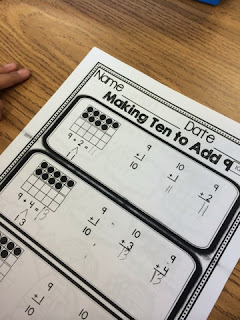Making Ten to Add is one of the most important concepts that early math learners must master.  It is one of the specific math strategies that is part of the operations and algebraic thinking strand of the Common Core State Math Standards: (1.OA.6a) (TEKS 1.3.D).
Add and subtract within 20, demonstrating fluency
for addition and subtraction within 10
(Addition and Subtraction Strategies).  Use strategies such as counting on and making ten. (e.g., 8 + 6 = 8 + 2 + 4 = 10 + 4 = 14).
This particular strategy is very important for your students to master in order to understand higher level math concepts.

Students “make ten” by breaking apart a smaller number and then adding the remainder of the broken-apart number to a ten.  Working with a ten in addition is much easier than working with any other number.  Making ten by breaking apart smaller numbers is crucial to understanding place value, its representation and the relationship between numbers.  All students need lots of practice with this skill in order to competently use it going forward.

Before mastering this concept, students learn how to make a ten in all of its different ways. (Common Core Math Standard 1.OA.3:  Properties of Operations).  For more on how I introduce the "TEN" click here.
Students needs lots of practice with new math skills.  It is imperative to understand how numbers may be "broken apart" to reach ten, then how to add numbers to ten.
During this time, I stress to students how very important ten is in our number system!
I introduce the "making ten" strategy during our carpet math time.  This is usually at the beginning of our math block (After Number of the Day).  I use this time to introduce math concepts in a group setting allowing my kiddos to lean on each other while I work with the whole group.
The TEKS alignment is 1.3.c (compose a ten) and 1.3.d (make a ten to add).
Making ten to subtract.

After I initially introduce the concept, we practice "making ten" in our math notebooks.  I use a document camera to project my math notebook, as students work at their desks in their our notebooks.I also use these pages for guided math work and intervention during teacher directed math at my desk.
Because students need so much practice when they learn a new math concept, I have introduced a new series of units that will give your students lots of practice with different math standards.  My Mega Math Practice units will have lots of practice with cool graphics to color when the practice is done.
Making Ten to Add: Holiday FREEBIE Edition  gives your students three pages of practice with  the math strategy “Making Ten to Add”.
Mega Math Practice: Making Ten to Add is aligned with the First Grade Common Core Math Standard 1.OA.6a.

The unit includes sixteen pages of practice with a holiday theme.  I use these pages during our “written math practice” rotation during math workshop/guided math time.  These pages can also be used for early finishers or homework during the time that you teach the 1.OA.6b math strategy. Watch for more MEGA MATH PRACTICE units to come aligned with the Common Core State Standards.
Mega Math Practice: Properties of Operations is aligned with the First Grade Common Core Math Standard 1.OA.3.

This unit includes twenty-six pages of practice with a winter theme.  These pages can also be used for early finishers or homework during the time that you teach the 1.OA.3.  Watch for more MEGA MATH PRACTICE units to come aligned with the Common Core State Standards.
I use ‘guided math’ in my classroom.  I begin my teaching time block teaching/spiraling math concepts as a whole group, but most of my math block is devoted to guided practice in small groups followed by independent or small group practice, centers and intervention.  I begin my math time every day with a page from my Number of the Day series.

I hope that you and your kids enjoy these fun holiday resources.